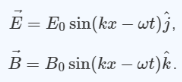# Problem: To understand the formula representing a traveling electromagnetic wave. Light, radiant heat (infrared radiation), X rays, and radio waves are all examples of traveling electromagnetic waves. Electromagnetic waves comprise combinations of electric and magnetic fields that are mutually compatible in the sense that the changes in one generate the other. The simplest form of a traveling electromagnetic wave is a plane wave. For a wave traveling in the x direction whose electric field is in the y direction, the electric and magnetic fields are given byThis wave is linearly polarized in the y direction.What is the wavelength λ of the wave described in the problem introduction?

###### FREE Expert Solution

Wave number, k, is defined as

$\overline{){\mathbf{k}}{\mathbf{=}}\frac{\mathbf{2}\mathbf{\pi }}{\mathbf{\lambda }}}$

In the wave equation, we already have k.

80% (148 ratings)###### Problem Details

To understand the formula representing a traveling electromagnetic wave. Light, radiant heat (infrared radiation), X rays, and radio waves are all examples of traveling electromagnetic waves. Electromagnetic waves comprise combinations of electric and magnetic fields that are mutually compatible in the sense that the changes in one generate the other. The simplest form of a traveling electromagnetic wave is a plane wave. For a wave traveling in the x direction whose electric field is in the y direction, the electric and magnetic fields are given byThis wave is linearly polarized in the y direction.

What is the wavelength λ of the wave described in the problem introduction?

Frequently Asked Questions

What scientific concept do you need to know in order to solve this problem?

Our tutors have indicated that to solve this problem you will need to apply the Electromagnetic Waves as Sinusoidal Waves concept. You can view video lessons to learn Electromagnetic Waves as Sinusoidal Waves. Or if you need more Electromagnetic Waves as Sinusoidal Waves practice, you can also practice Electromagnetic Waves as Sinusoidal Waves practice problems.